# What can be said about an Endothermic reaction with a negative entropy change?

What can be said about an Endothermic reaction with a negative entropy change?

The reaction is
1. spontaneous at all temperatures.
2. spontaneous at high temperatures.
3. spontaneous at low temperatures.
4. spontaneous in the reverse direction at all temperatures.
5. nonspontaneous in either direction at all temperatures.

What can be said about an Exothermic reaction with a negative entropy change?

The reaction is
1. spontaneous at all temperatures.
2. spontaneous at high temperatures.
3. spontaneous at low temperatures.
4. spontaneous in the reverse direction at all temperatures.
5. nonspontaneous in either direction at all temperatures.

Concepts and reason
The concepts used to solve this question are the characteristics of endothermic and exothermic reactions, and the Gibbs free energy equation to determine a spontaneous reaction.

Fundamentals
The change in Gibb’s free energy at constant temperature and pressure is given as;

Enthalpy (H) in thermodynamics is defined as the heat content of a reaction. Entropy (S) is termed as the quantification of disorder or randomness of a reaction. Gibb’s free energy change helps to determine the direction of the reaction.

Part 1
The question states that it is an endothermic reaction with negative entropy. This means that the change in enthalpy will be positive and change in entropy will be negative. That is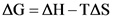An endothermic reaction generally requires energy from the surroundings for it to proceed. The energy absorbed is usually in the form of heat. Therefore, the change in enthalpy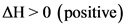of an endothermic reaction will always be positive. A negative change in entropy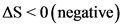indicates that there is a decrease in disorder, with respect to the reaction.

The change in Gibb’s free energy at constant temperature and pressure is given as-Now, the change in enthalpy and change in entropy can be written as follows,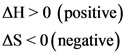Substitute these values in the above equation;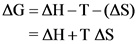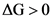,thus, the reaction is spontaneous in the reverse direction at all temperatures.

Part 1
The reaction is spontaneous in the reverse direction at all temperatures.

One can make a mistake while substituting the signs in the Gibb’s equation. This in turn results in a miscalculation of the final answer. This can be avoided by keeping in mind the given conditions of the reaction and very attentively substituting the signs of the values.

Part 2
The question states that it is an exothermic reaction with negative entropy. This means that both the change in enthalpy and change in entropy will be negative. That is;An exothermic reaction generally releases energy to the surroundings. The energy is usually released in the form of heat. Therefore, the change in enthalpy H of an exothermic reaction will always be negative. A negative change in entropy S indicates that there is a decrease in disorder, with respect to the reaction.

The change in Gibb’s free energy at constant temperature and pressure is given as-Now, the change in enthalpy and change in entropy can be written as follows,Substitute these values in the above equation;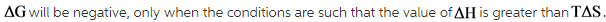Part 2
The reaction is spontaneous at low temperatures.

According to the sign convention in this equation,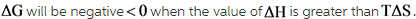This can occur only at low temperatures (T has small values). Thus, this reaction is spontaneous at low temperatures.

Part 1
The reaction is spontaneous in the reverse direction at all temperatures.

Part 2
The reaction is spontaneous at low temperatures.